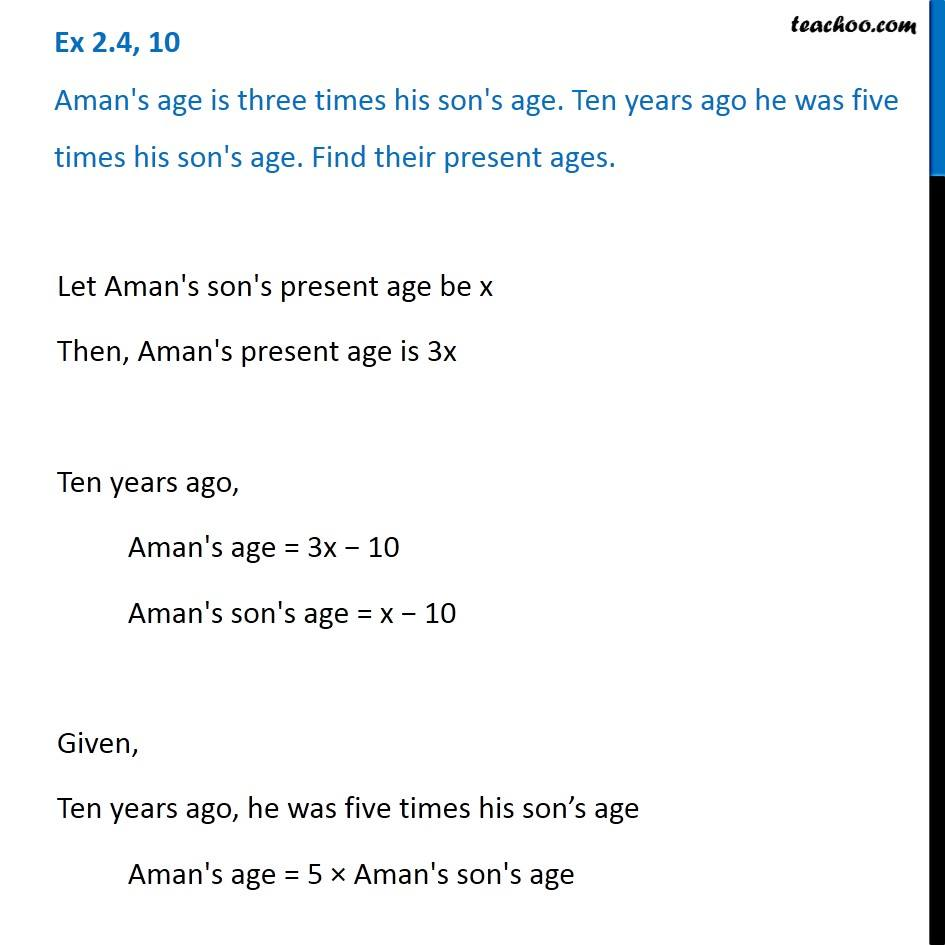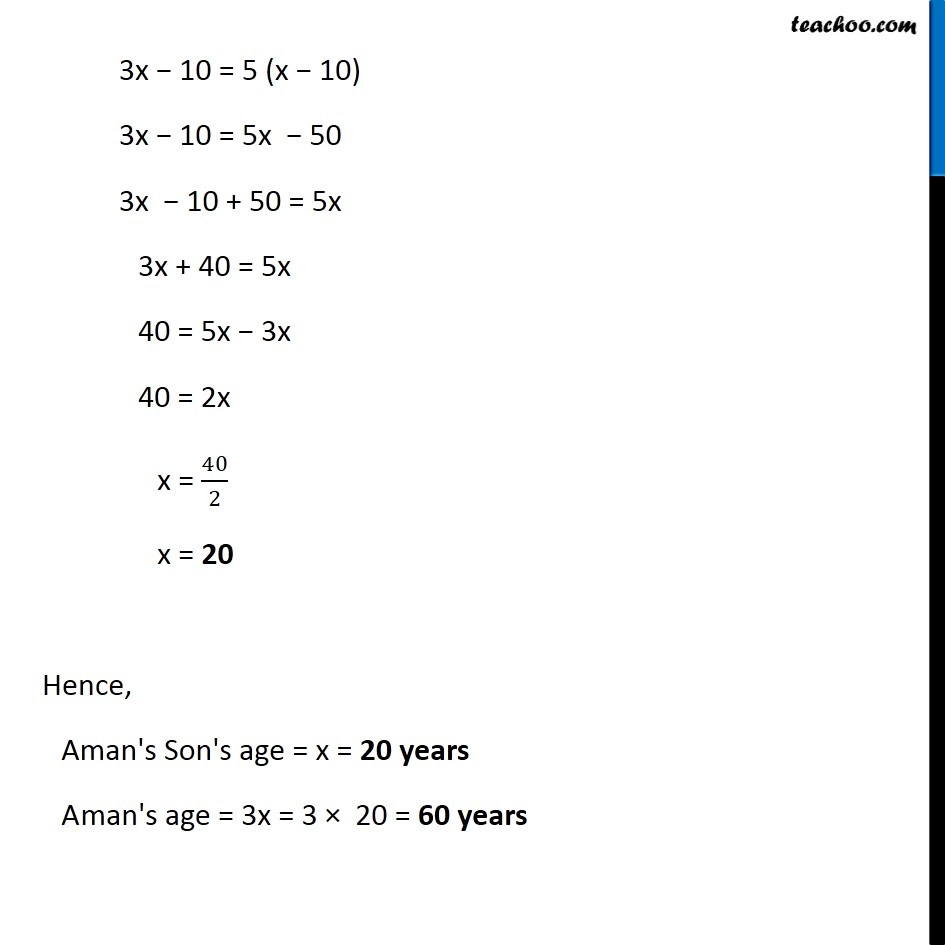Solving difficult equations

Chapter 2 Class 8 Linear Equations in One Variable
Serial order wiseLearn in your speed, with individual attention - Teachoo Maths 1-on-1 Class

### Transcript

Question 10 Aman's age is three times his son's age. Ten years ago he was five times his son's age. Find their present ages.Let Aman's son's present age be x Then, Aman's present age is 3x Ten years ago, Aman's age = 3x 10 Aman's son's age = x 10 Given, Ten years ago, he was five times his son s age Aman's age = 5 Aman's son's age 3x 10 = 5 (x 10) 3x 10 = 5x 50 3x 10 + 50 = 5x 3x + 40 = 5x 40 = 5x 3x 40 = 2x x = 40/2 x = 20 Hence, Aman's Son's age = x = 20 years A man's age = 3x = 3 20 = 60 years